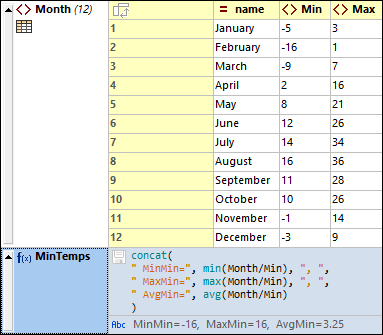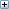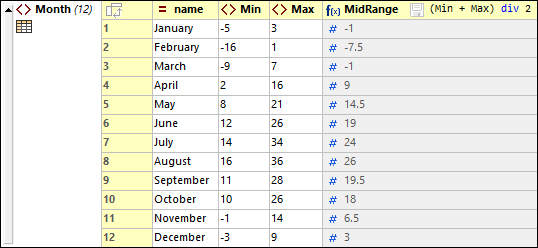# Altova XMLSpy 2022 Professional Edition

 Formulas Scroll Home Prev Top Next More

A formula in XML Grid View uses an XQuery 3.1 expression to calculate a result or generate a nodeset that can be stored in the document. A formula is defined with an XQuery 3.1 expression. For example, in the screenshot below, a formula named MinTemps has been created that generates the minimum, maximum, and average of the set of all minimum temperatures.To create a formula, do the following:

1.Add a new node where you want to display the formula.

2.The node will be created by default as an element. Change the type of the node to Formula.

3.Double-click in the cell containing the f(x) icon and enter the name of the formula (see screenshot above).

4.You can click this icon to save the output of the formula to the document.

5.Double-click in the expression's cell and type in the expression, then click Enter.Listing of XML document used in the screenshot aboveListing of formula expression used in the screenshot above

Note the following points:

The context node of the formula's XQuery expression is the parent node of the formula node.

To add a new line in an expression, press Ctrl+Enter. This is useful if you want to display an expression over several lines for better readability.

Formulas (that is, the expressions defining formulas) are stored in the metadata of the document (located in a application-wide metadata file). Formulas are also saved as processing instructions in the XML file.

The result generated by a formula is displayed in the cell below the formula's XQuery expression and stored in the document's metadata. The result can also be stored in the document. Do this by clicking the disk icon next to the XQuery expression. Whether the icon is clicked or not, the formula's result will be calculated and stored in the document's metadata.

When the formula result is a calculation, it is stored as content of an element which has the name you assigned the formula. For example, in the MinTemps formula shown in the screenshot above, the result will be stored in an element named MinTemps.

Go to to: (i) specify whether to store formulas in metadata always, only on request, or never, (ii) specify whether to store formulas in the document.

## Formulas in tables

If all the cells of a table column (in Table Display) contain the same formula, then the formula is displayed only once—in the header of the column (see screenshot below). The results of the formula calculation, however, are displayed in the respective cells. The formula in the column header is a Grid View representation. In the XML document (see Text View), the formula is repeated for each table-row item.If even a single formula of a cell is different, then each formula is displayed in its respective cell. If all formulas of a table column are the same so that the formula appears in the header, and you now want to create a different formula for an individual cell, switch off Table Display and edit the formula of that cell. When you switch back to Table Display, formulas will be displayed in individual cells (for all cells).